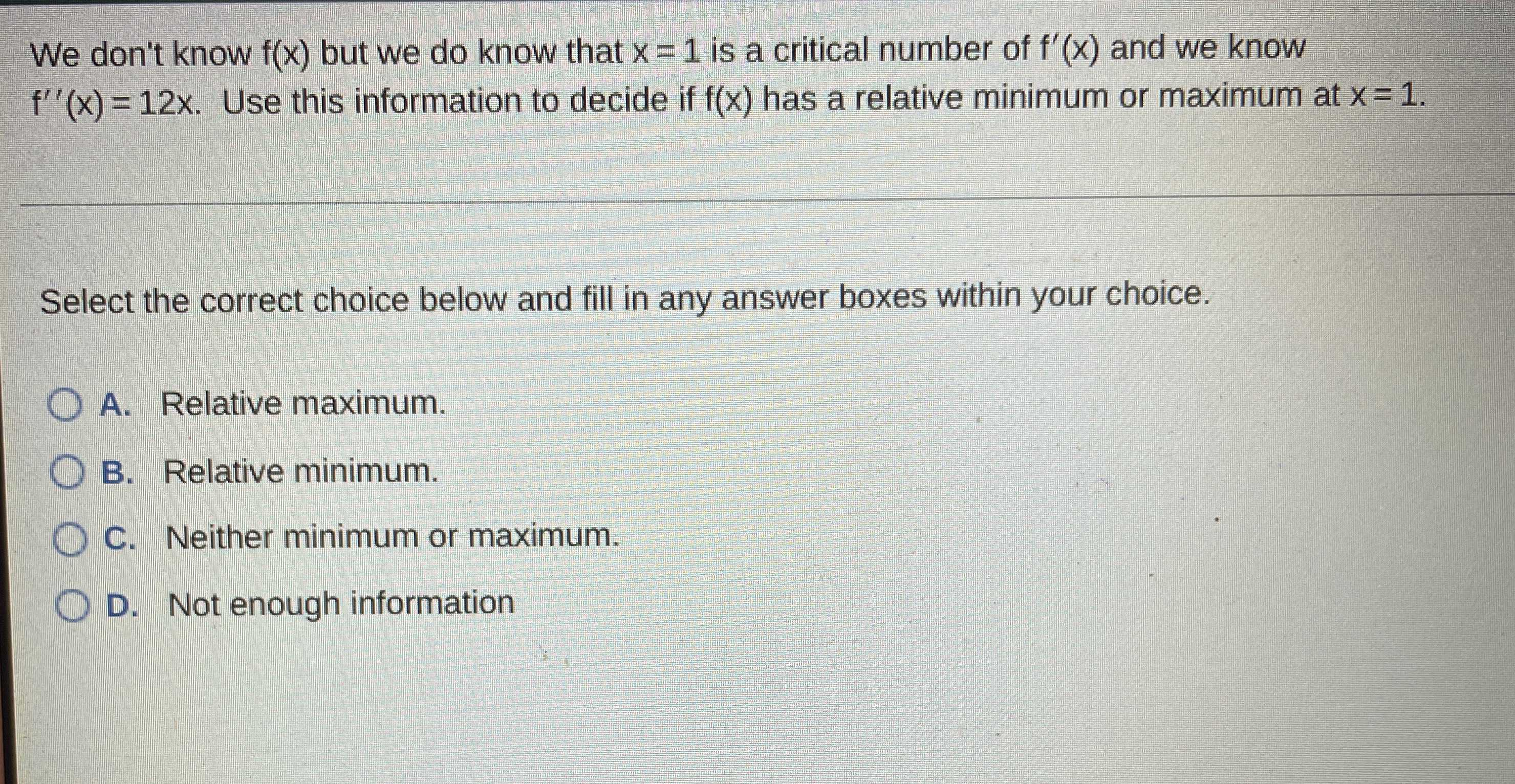### Still have math questions?

Algebra
QuestionWe don't know $$f ( x )$$ but we do know that $$x = 1$$ is a critical number of $$f ^ { \prime } ( x )$$ and we know $$f ^ { \prime \prime } ( x ) = 12 x$$ . Use this information to decide if $$f ( x )$$ has a relative minimum or maximum at $$x = 1$$

Select the correct choice below and fill in any answer boxes within your choice.

A. Relative maximum.

B. Relative minimum.

C. Neither minimum or maximum.

D. Not enough information